详解 Kaggle 房价预测竞赛优胜方案：用 Python 进行全面数据探索

Kaggle 的房价预测竞赛从 2016 年 8 月开始，到 2017 年 2 月结束。这段时间内，超过 2000 多人参与比赛，选手采用高级回归技术，基于我们给出的 79 个特征，对房屋的售价进行了准确的预测。今天我们介绍的是目前得票数最高的优胜方案：《用 Python 进行全面数据探索》，该方案在数据探索，特征工程上都有十分出色的表现。

1. 定义要解决的问题；

2. 查阅相关文献；

3. 对他们进行修改以适合自己的要求。

了解你的数据

• 理解问题：查看每个变量并且根据他们的意义和对问题的重要性进行哲学分析。

• 单因素研究：只关注因变量( SalePrice)，并且进行更深入的了解。

• 多因素研究：分析因变量和自变量之间的关系。

• 基础清洗：清洗数据集并且对缺失数据，异常值和分类数据进行一些处理。

• 检验假设：检查数据是否和多元分析方法的假设达到一致。

import pandas as pd
import matplotlib.pyplot as plt
import seaborn as sns
import numpy as npfrom scipy.stats
import normfrom sklearn.preprocessing
import StandardScalerfrom scipy
import stats
import warnings
warnings.filterwarnings('ignore')
%matplotlib inline

#bring in the six packs
#check the decoration
df_train.columns

Index(['Id', 'MSSubClass', 'MSZoning','LotFrontage', 'LotArea', 'Street',
'Alley', 'LotShape', 'LandContour', 'Utilities','LotConfig',
'LandSlope', 'Neighborhood', 'Condition1','Condition2', 'BldgType',
'RoofStyle', 'RoofMatl', 'Exterior1st','Exterior2nd', 'MasVnrType',
'MasVnrArea', 'ExterQual', 'ExterCond','Foundation', 'BsmtQual',
'BsmtCond', 'BsmtExposure', 'BsmtFinType1','BsmtFinSF1',
'BsmtFinType2', 'BsmtFinSF2', 'BsmtUnfSF','TotalBsmtSF', 'Heating',
'HeatingQC', 'CentralAir', 'Electrical', '1stFlrSF','2ndFlrSF',
'LowQualFinSF', 'GrLivArea', 'BsmtFullBath','BsmtHalfBath', 'FullBath',
'HalfBath', 'BedroomAbvGr', 'KitchenAbvGr','KitchenQual',
'TotRmsAbvGrd', 'Functional', 'Fireplaces','FireplaceQu', 'GarageType',
'GarageYrBlt', 'GarageFinish', 'GarageCars','GarageArea', 'GarageQual',
'GarageCond', 'PavedDrive', 'WoodDeckSF','OpenPorchSF',
'EnclosedPorch', '3SsnPorch', 'ScreenPorch','PoolArea', 'PoolQC',
'Fence', 'MiscFeature', 'MiscVal', 'MoSold','YrSold', 'SaleType',
'SaleCondition', 'SalePrice'],
dtype='object')

• 变量 – 变量名。

• 类型 – 该变量的类型。这一栏只有两个可能值，“数据” 或 “类别”。 “数据” 是指该变量的值是数字，“类别” 指该变量的值是类别标签。

• 划分 – 指示变量划分. 我们定义了三种划分：建筑，空间，位置。

• 期望 – 我们希望该变量对房价的影响程度。我们使用类别标签 “高”，“中” 和 “低” 作为可能值。

• 结论 – 我们得出的该变量的重要性的结论。在大概浏览数据之后，我们认为这一栏和 “期望” 的值基本一致。

• 评论 – 我们看到的所有一般性评论。

• 我们买房子的时候会考虑这个因素吗？

• 如果考虑的话，这个因素的重要程度如何？

• 这个因素带来的信息在其他因素中出现过吗？

• OverallQual

• YearBuilt.

• TotalBsmtSF.

• GrLivArea.

描述性数据总结：

df_train['SalePrice'].describe()

count      1460.000000
mean     180921.195890
std       79442.502883
min       34900.000000
25%      129975.000000
50%      163000.000000
75%      214000.000000
max      755000.000000
Name: SalePrice, dtype: float64

绘制直方图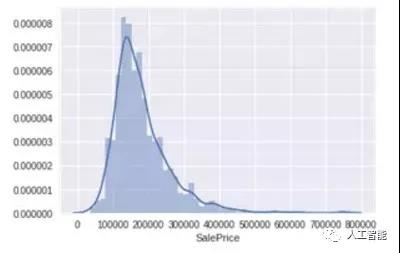sns.distplot(df_train['SalePrice']);

• 偏离正态分布

• 数据正偏

• 有峰值

数据偏度和峰度度量：

print("Skewness: %f" % df_train['SalePrice'].skew())
print("Kurtosis: %f" % df_train['SalePrice'].kurt())

Skewness: 1.882876

Kurtosis: 6.536282

“房价” 的相关变量分析

1. Grlivarea 与 SalePrice 散点图

var = 'GrLivArea'
data = pd.concat([df_train['SalePrice'], df_train[var]], axis=1)
data.plot.scatter(x=var, y='SalePrice', ylim=(0,800000));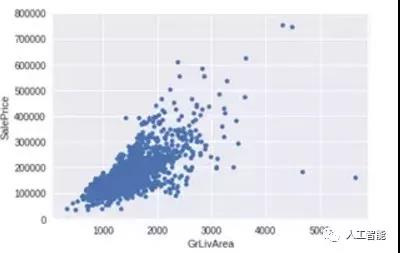2. TotalBsmtSF 与 SalePrice 散点图

var = 'TotalBsmtSF'
data = pd.concat([df_train['SalePrice'], df_train[var]], axis=1)
data.plot.scatter(x=var, y='SalePrice', ylim=(0,800000));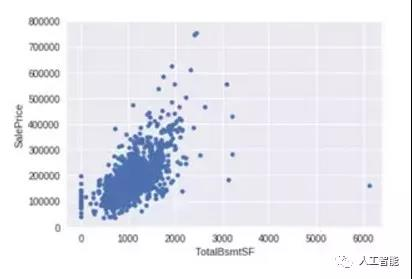TotalBsmtSF  和 SalePrice 关系也很密切，从图中可以看出基本呈指数分布，但从最左侧的点可以看出特定情况下 TotalBsmtSF 对 SalePrice 没有产生影响。

与类别型变量的关系

1.‘OverallQual’与‘SalePrice’箱型图

var = 'OverallQual'
data = pd.concat([df_train['SalePrice'], df_train[var]], axis=1)
f, ax = plt.subplots(figsize=(8, 6))
fig = sns.boxplot(x=var, y="SalePrice", data=data)
fig.axis(ymin=0, ymax=800000);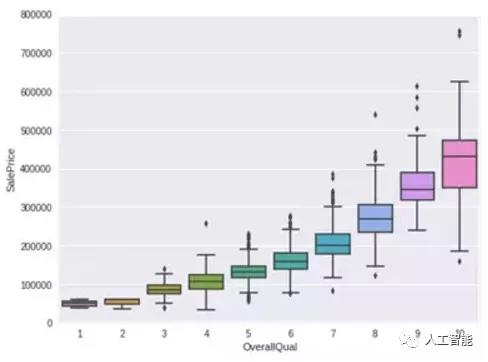2. YearBuilt 与 SalePrice 箱型图

var = 'YearBuilt'
data = pd.concat([df_train['SalePrice'], df_train[var]], axis=1)
f, ax = plt.subplots(figsize=(16, 8))
fig = sns.boxplot(x=var, y="SalePrice", data=data)
fig.axis(ymin=0, ymax=800000);plt.xticks(rotation=90);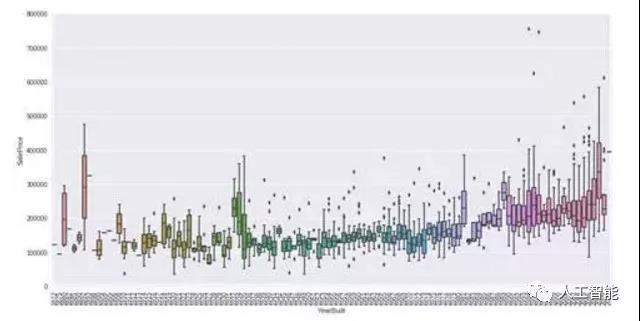• GrLivArea 和 TotalBsmtSF 与 SalePrice 似乎线性相关，并且都是正相关。 对于 TotalBsmtSF 线性关系的斜率十分的高。

• OverallQual 和 YearBuilt 与 SalePrice 也有关系。OverallQual 的相关性更强, 箱型图显示了随着整体质量的增长，房价的增长趋势。

客观分析

1. 相关系数矩阵

corrmat = df_train.corr()
f, ax = plt.subplots(figsize=(12, 9))
sns.heatmap(corrmat, vmax=.8, square=True);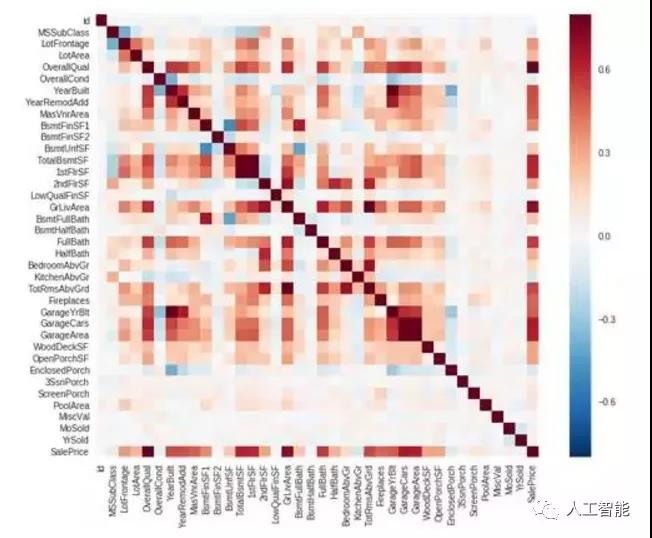2. SalePrice 相关系数矩阵

k = 10 #number ofvariables for heatmap
cols = corrmat.nlargest(k, 'SalePrice')['SalePrice'].index
cm = np.corrcoef(df_train[cols].values.T)
sns.set(font_scale=1.25)
hm = sns.heatmap(cm, cbar=True, annot=True, square=True, fmt='.2f', annot_kws={'size': 10},
yticklabels=cols.values, xticklabels=cols.values)
plt.show()• OverallQual，GrLivArea 以及 TotalBsmtSF  与 SalePrice 有很强的相关性。

• GarageCars 和 GarageArea 也是相关性比较强的变量. 车库中存储的车的数量是由车库的面积决定的，它们就像双胞胎，所以不需要专门区分 GarageCars 和 GarageAre，所以我们只需要其中的一个变量。这里我们选择了 GarageCars，因为它与 SalePrice 的相关性更高一些。

• TotalBsmtSF  和 1stFloor 与上述情况相同，我们选择 TotalBsmtS 。

• FullBath 几乎不需要考虑。

• TotRmsAbvGrd 和 GrLivArea 也是变量中的双胞胎。

• YearBuilt 和 SalePrice 相关性似乎不强。

3. SalePrice 和相关变量之间的散点图

sns.set()
cols = ['SalePrice', 'OverallQual', 'GrLivArea','GarageCars', 'TotalBsmtSF', 'FullBath', 'YearBuilt']
sns.pairplot(df_train[cols], size = 2.5)
plt.show();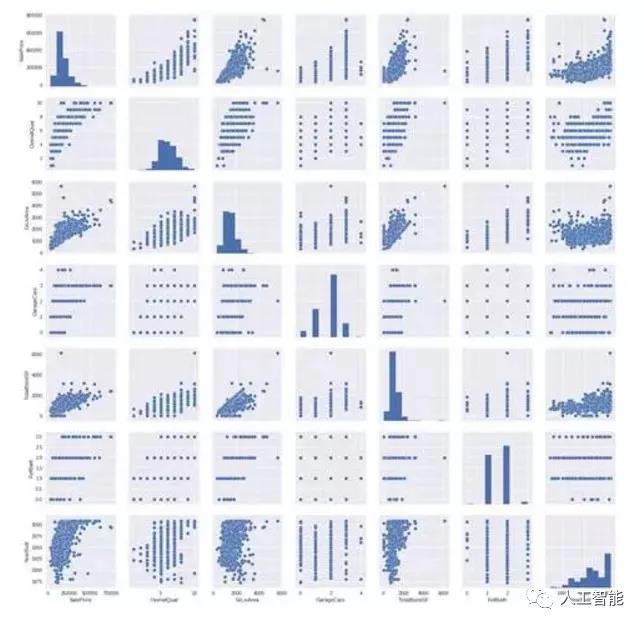SalePrice 和 YearBuilt 之间的散点图也值得我们思考。在 “点云” 的底部，我们可以观察到一个几乎呈指数函数的分布。我们也可以看到 “点云” 的上端也基本呈同样的分布趋势。并且可以注意到，近几年的点有超过这个上端的趋势。

缺失数据

• 这一缺失数据的普遍性如何？

• 缺失数据是随机的还是有律可循？

total= df_train.isnull().sum().sort_values(ascending=False)
percent = (df_train.isnull().sum()/df_train.isnull().count()).sort_values(ascending=False)
missing_data = pd.concat([total, percent], axis=1, keys=['Total','Percent'])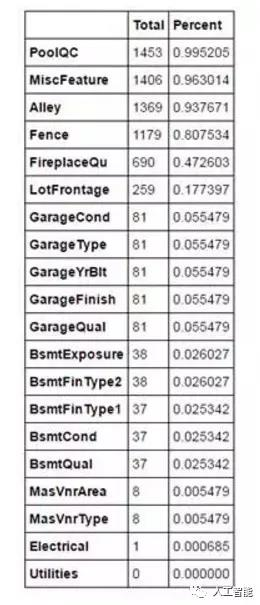df_train= df_train.drop((missing_data[missing_data['Total'] > 1]).index,1)
df_train= df_train.drop(df_train.loc[df_train['Electrical'].isnull()].index)
df_train.isnull().sum().max() #justchecking that there's no missing data missing...

异常值

saleprice_scaled= StandardScaler().fit_transform(df_train['SalePrice'][:,np.newaxis]);
low_range = saleprice_scaled[saleprice_scaled[:,0].argsort()][:10]
high_range= saleprice_scaled[saleprice_scaled[:,0].argsort()][-10:]
print('outer range (low) of the distribution:')
print(low_range)
print('\nouter range (high) of thedistribution:')
print(high_range)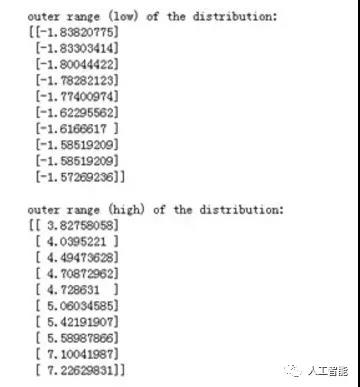• 低范围的值都比较相似并且在 0 附近分布。

• 高范围的值离 0 很远，并且七点几的值远在正常范围之外。

双变量分析

1.  GrLivArea 和 SalePrice 双变量分析

var = 'GrLivArea'
data = pd.concat([df_train['SalePrice'], df_train[var]], axis=1)
data.plot.scatter(x=var, y='SalePrice', ylim=(0,800000));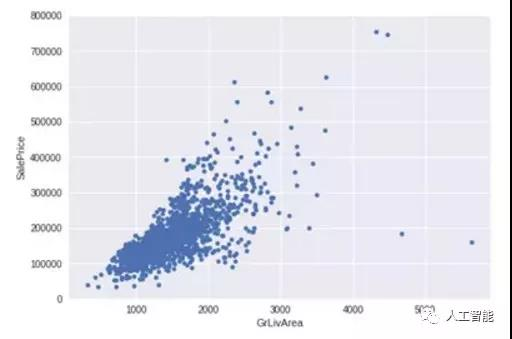• 有两个离群的 GrLivArea 值很高的数据，我们可以推测出现这种情况的原因。或许他们代表了农业地区，也就解释了低价。 这两个点很明显不能代表典型样例，所以我们将它们定义为异常值并删除。

• 图中顶部的两个点是七点几的观测值，他们虽然看起来像特殊情况，但是他们依然符合整体趋势，所以我们将其保留下来。

df_train.sort_values(by = 'GrLivArea',ascending = False)[:2]
df_train = df_train.drop(df_train[df_train['Id'] == 1299].index)
df_train = df_train.drop(df_train[df_train['Id'] == 524].index)

2.  TotalBsmtSF 和 SalePrice 双变量分析

var = 'TotalBsmtSF'
data = pd.concat([df_train['SalePrice'],df_train[var]], axis=1)
data.plot.scatter(x=var, y='SalePrice',ylim=(0,800000));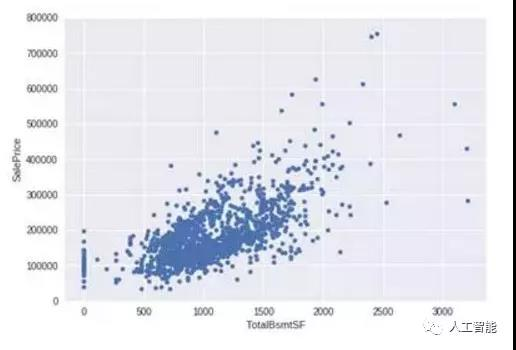核心部分

“房价” 到底是谁？

• 正态性

• 同方差性

• 线性

• 相关错误缺失

• 直方图 – 峰度和偏度。

• 正态概率图 – 数据分布应紧密跟随代表正态分布的对角线。

1.  SalePrice

sns.distplot(df_train['SalePrice'], fit=norm);
fig = plt.figure()
res = stats.probplot(df_train['SalePrice'], plot=plt)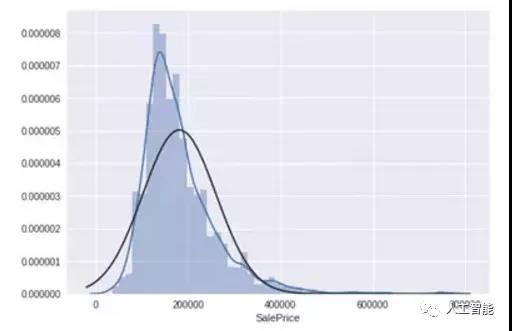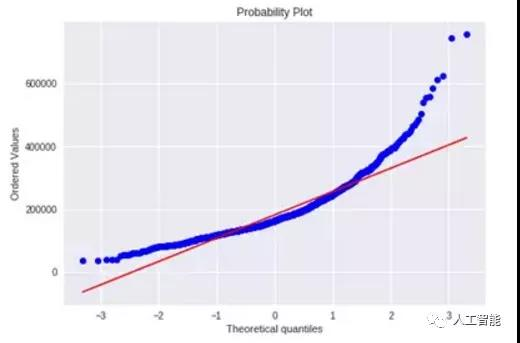df_train['SalePrice']= np.log(df_train['SalePrice'])

sns.distplot(df_train['SalePrice'], fit=norm);
fig = plt.figure()
res = stats.probplot(df_train['SalePrice'], plot=plt)

2. GrLivArea

sns.distplot(df_train['GrLivArea'], fit=norm);
fig = plt.figure()
res = stats.probplot(df_train['GrLivArea'], plot=plt)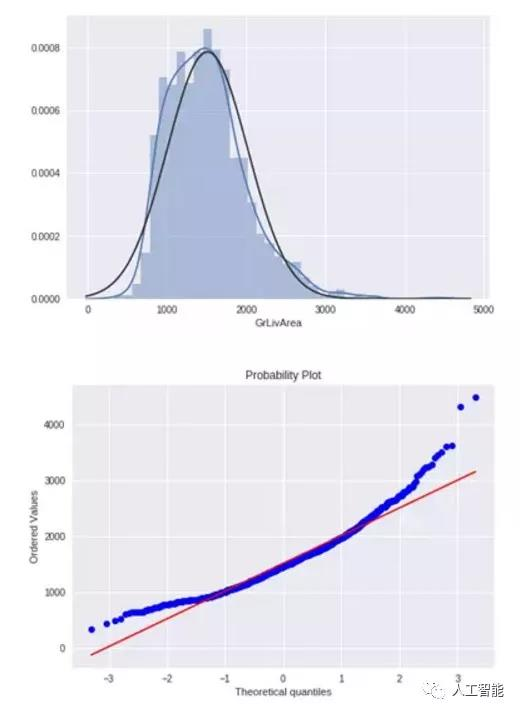df_train['GrLivArea']= np.log(df_train['GrLivArea'])

sns.distplot(df_train['GrLivArea'], fit=norm);
fig = plt.figure()
res = stats.probplot(df_train['GrLivArea'], plot=plt)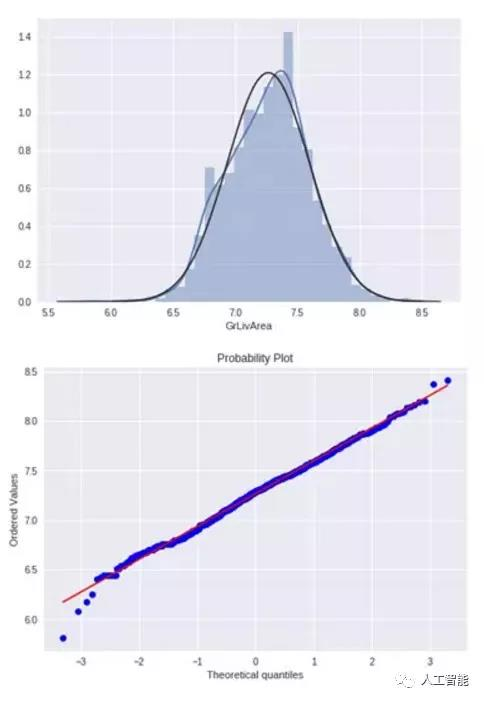3.  TotalBsmtSF

sns.distplot(df_train['TotalBsmtSF'],fit=norm);
fig = plt.figure()
res = stats.probplot(df_train['TotalBsmtSF'],plot=plt)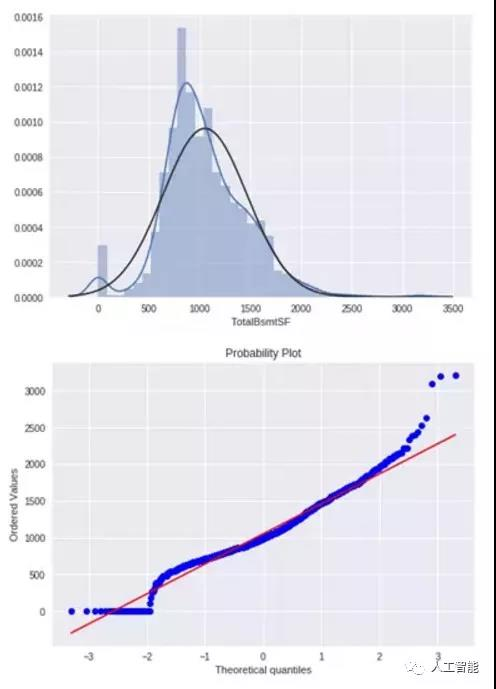• 显示出了偏度

• 大量为 0 的观察值（没有地下室的房屋）

• 含 0 的数据无法进行对数变换

df_train['HasBsmt']= pd.Series(len(df_train['TotalBsmtSF']), index=df_train.index)
df_train['HasBsmt'] = 0
df_train.loc[df_train['TotalBsmtSF']>0,'HasBsmt'] = 1

df_train['TotalBsmtSF']= np.log(df_train['TotalBsmtSF'])

sns.distplot(df_train['TotalBsmtSF'], fit=norm);
fig = plt.figure()
res = stats.probplot(df_train['TotalBsmtSF'], plot=plt)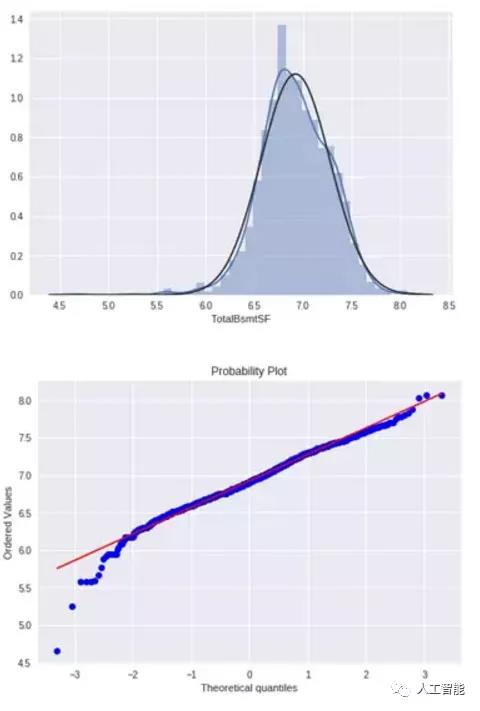1.  SalePrice 和 GrLivArea 同方差性

plt.scatter(df_train['GrLivArea'],df_train['SalePrice']);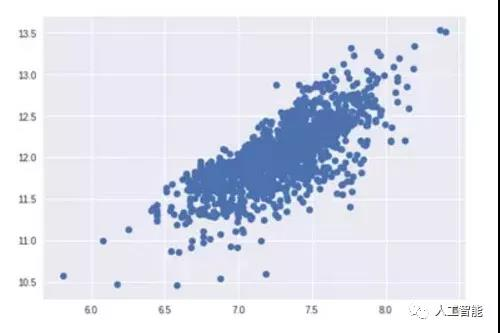2. SalePrice with TotalBsmtSF 同方差性

plt.scatter(df_train[df_train['TotalBsmtSF']>0]
['TotalBsmtSF'], df_train[df_train['TotalBsmtSF']>0]['SalePrice']);虚拟变量

df_train = pd.get_dummies(df_train)